Next: Wavefunction Collapse Up: Wave Mechanics Previous: Wave Packets

# Heisenberg's Uncertainty Principle

According to the analysis contained in the previous section, a particle wave packet that is initially localized in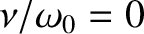-space, with characteristic width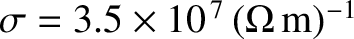, is also localized in-space, with characteristic width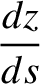. However, as time progresses, the width of the wave packet in-space increases [see Equation (1133)], while that of the packet in-space stays the same [because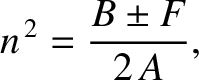is given by Equation (1123) at all times]. Hence, in general, we can say that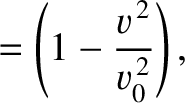(1134)

Furthermore, we can interpretand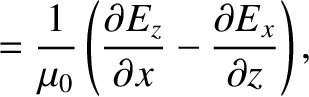as characterizing our uncertainty regarding the values of the particle's position and wavenumber, respectively.

A measurement of a particle's wavenumber,, is equivalent to a measurement of its momentum,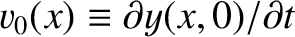, because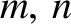. Hence, an uncertainty inof ordertranslates to an uncertainty inof order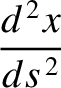. It follows, from the previous inequality, that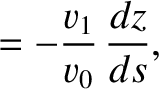(1135)

This is the famous Heisenberg uncertainty principle, first proposed by Werner Heisenberg in 1927 (Dirac 1982). According to this principle, it is impossible to simultaneously measure the position and momentum of a particle (exactly). Indeed, a good knowledge of the particle's position implies a poor knowledge of its momentum, and vice versa. The uncertainty principle is a direct consequence of representing particles as waves.

It is apparent, from Equation (1133), that a particle wave packet of initial spatial extent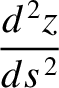spreads out in such a manner that its spatial extent becomes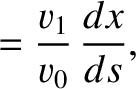(1136)

at large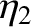. It is readily demonstrated that this spreading of the wave packet is a consequence of the uncertainty principle. Indeed, because the initial uncertainty in the particle's position is, it follows that the uncertainty in its momentum is of order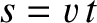. This translates to an uncertainty in velocity of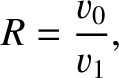. Thus, if we imagine that part of the wave packet propagates at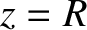, and another part at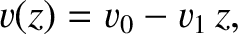, where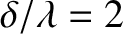is the mean propagation velocity, then it follows that the wave packet will spread out as time progresses. Indeed, at large, we expect the width of the wave packet to be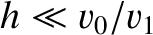(1137)

which is identical to Equation (1136). Evidently, the spreading of a particle wave packet, as time progresses, should be interpreted as representing an increase in our uncertainty regarding the particle's position, rather than an increase in the spatial extent of the particle itself.Next: Wavefunction Collapse Up: Wave Mechanics Previous: Wave Packets
Richard Fitzpatrick 2013-04-08